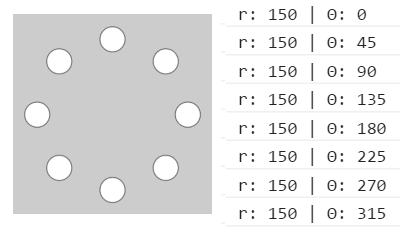# Problem plotting points

Hey gang,

I am trying to plot some points onto a canvas. I’ve made a polar to cartesian function. But I’m getting some unexpected results. Could anyone tell me what I’m doing wrong here?

``````var c = document.getElementById("myCanvas");
var cxt = c.getContext("2d");

var centerX = 75;
var centerY = 75;
const r = 3;
const col = "#6f0c4f";

//draw the center point:
drawCirc(centerX, centerY, r, col);

function drawCirc(x, y, radius, stroke) {
cxt.beginPath();
cxt.arc(x, y, radius, 0, 2 * Math.PI);
cxt.strokeStyle = stroke;
cxt.stroke();
cxt.fillStyle = stroke;
cxt.fill();
}

var pointsArray = [];

function polarToCartesian(r, theta) {
const x = r * Math.cos(theta) + 75;
const y = r * Math.sin(theta) + 75;

return pointsArray.push({
x: x,
y: y,
})
}

polarToCartesian(150, 22.5)
polarToCartesian(150, 45)
polarToCartesian(150, 67.5)
polarToCartesian(150, 90)
polarToCartesian(150, 112.5)
polarToCartesian(150, 135)
polarToCartesian(150, 157.5)
polarToCartesian(150, 202.5)
polarToCartesian(150, 225)
polarToCartesian(150, 247.5)
polarToCartesian(150, 270)
polarToCartesian(150, 292.5)
polarToCartesian(150, 315)
polarToCartesian(150, 337.5)
polarToCartesian(150, 360)

pointsArray.forEach((p) => {
console.log(p)
drawCirc(p.x, p.y, r, col)
})

``````

https://codepen.io/edubz/pen/yLgRYXq

Hello there,

What are you expecting to get?

1 LikeRight.

This is an easy fix:

1. Do not use hardcoded values like: `centerX`
• You have access to the canvas (`centerX` is the canvas width divided by 2)
1. This formula is almost correct, but, from its order, it looks like you might be thinking about the execution in difficult way:
``````r * Math.cos(theta) + 75;
``````
• What you want to do is:
• Take the center of your “circle” (based on the center of the canvas)
• Add the radius @ some angle

If this does not make much sense…sorry, but here is the formula:

``````x = offset + r X cos(theta)
``````

Now, to optomise your code (if you are interested):

• I would not calculate the offset within the `polar2Cartesian` function, because, what if you want to use a different center of circle?
• The minute you see code like below, think how to DRY (don’t repeat yourself) it out:
``````polarToCartesian(150, 22.5)
polarToCartesian(150, 45)
polarToCartesian(150, 67.5)
...
``````
• What is repeated, and how are these points calculated?

EDIT: I helped someone with something similar, and decided to take it further, if you are interested: Moving drawn ctx image - #3 by Sky020

Hope this helps

1 Like

you bring up some good points @Sky020. For some reason tho that formula wasnt really clicking for me. So I went the long way around. After some reading and tinkering I was able to piece together a working draft https://codepen.io/edubz/pen/yLgRYXq?editors=0011.

It is based off of the functions in the patterns section of this article https://varun.ca/polar-coords/

This topic was automatically closed 182 days after the last reply. New replies are no longer allowed.